Download PresentationAnnouncements

# Announcements - PowerPoint PPT Presentation

Homework – Chapter 4 8, 11, 13, 17, 19, 22 Chapter 6 6, 9, 14, 15. Exam Thursday. Announcements. 4-8. Meaning of Confidence interval? Is an interval around the experimental mean that most likely contains the true mean ( m ). Homework. 4.11. Question 4-13.I am the owner, or an agent authorized to act on behalf of the owner, of the copyrighted work described.
Download Presentation## Announcements

An Image/Link below is provided (as is) to download presentation

Download Policy: Content on the Website is provided to you AS IS for your information and personal use and may not be sold / licensed / shared on other websites without getting consent from its author.While downloading, if for some reason you are not able to download a presentation, the publisher may have deleted the file from their server.

- - - - - - - - - - - - - - - - - - - - - - - - - - E N D - - - - - - - - - - - - - - - - - - - - - - - - - -
Presentation TranscriptHomework –

Chapter 4

8, 11, 13, 17, 19, 22

Chapter 6

6, 9, 14, 15

Exam

Thursday

Announcements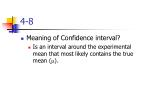4-8
• Meaning of Confidence interval?
• Is an interval around the experimental mean that most likely contains the true mean (m).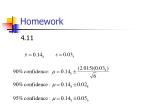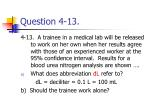Question 4-13.

4-13. A trainee in a medical lab will be released to work on her own when her results agree with those of an experienced worker at the 95% confidence interval. Results for a blood urea nitrogen analysis are shown ….

• What does abbreviation dL refer to?

dL = deciliter = 0.1 L = 100 mL

b) Should the trainee work alone?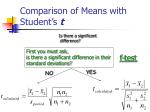Comparison of Means with Student’s t

Is there a significant

difference?

First you must ask,

is there a significant difference in their standard deviations?

f-test

YES

NO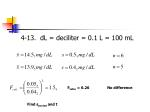4-13. dL = deciliter = 0.1 L = 100 mL

Ftable = 6.26

No difference

Find spooled and tttable = 2.262

No significant difference between two workers …

Therefore trainee should be “Released”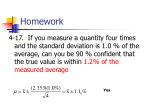Homework

4-17. If you measure a quantity four times and the standard deviation is 1.0 % of the average, can you be 90 % confident that the true value is within 1.2% of the measured average

Yes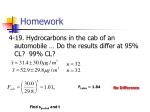Homework

4-19. Hydrocarbons in the cab of an automobile … Do the results differ at 95% CL? 99% CL?

Ftable ~ 1.84

No Difference

Find spooled and t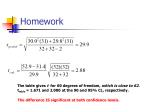Homework

The table gives t for 60 degrees of freedom, which is close to 62.

ttable = 1.671 and 2.000 at the 90 and 95% CL, respectively.

The difference IS significant at both confidence levels.4-22. Q-test, Is 216 rejectable?
• 192, 216, 202, 195, 204

Qtable = 0.64

Retain the “outlier” 216### Chapter 6

Chemical EquilibriumChemical Equilibrium
• Equilibrium Constant
• Equilibrium and Thermodynamics
• Enthalpy
• Entropy
• Free Energy
• Le Chatelier’s Principle
• Solubility product (Ksp)
• Common Ion Effect
• Separation by precipitation
• Complex formationNH3 + H2O NH4+ + OH-

Find the Equilibrium constant for the following reaction

NH4+ NH3 + H+

Example

The equilibrium constant for the reaction

H2O H+ + OH-

Kw = 1.0 x 10-14

KNH3 = 1.8 x 10-5

K3 = ?### Equilibrium and Thermodynamics

A brief review …Equilibrium and Thermodynamics

enthalpy => H

enthalpy change =>DH

exothermic vs. endothermic

entropy => S

free energy

Gibbs free energy => G

Gibbs free energy change =>DGEquilibrium and Thermodynamics

DGo =DHo - TDSo

DGo= -RT ln (K)

K = e-(DGo/RT)Equilibrium and Thermodynamics
• The case of HCl

HCl H+ + Cl-

K=?

DHo = -74.83 x 103 J/mol

DS0 = -130.4 kJ/mol

DGo =DHo - TDSo

DGo = (-74.83 kJ/mol) – (298.15 K) (-130.4 kJ/mol)

DGo = -35.97 kJ/molEquilibrium and Thermodynamics
• The case of HCl

HCl H+ + Cl-

K=?

DGo = (-74.83 kJ/mol) – (298.15 K) (-130.4 kJ/mol)

DGo = -35.97 kJ/mol### Predicting the direction in which an equilibrium will initially move

LeChatelier’s Principle and Reaction QuotientLe Chatelier's Principle
• If a stress, such as a change in concentration, pressure, temperature, etc., is applied to a system at equilibrium, the equilibrium will shift in such a way as to lessen the effect of the stress.
• Stresses –
• Adding or removing reactants or products
• Changing system equilibrium temperature
• Changing pressure (depends on how the change is accomplishedEquilibrium moves Right

Consider

6CO2 (g) + 6 H2O(g) C6H12O6(s) + 6O2(g)

Predict in which direction the equilibrium moves as a result of the following stress:

Increasing [CO2]Equilibrium moves Left

Consider

6CO2 (g) + 6 H2O(g) C6H12O6(s) + 6O2(g)

Predict in which direction the equilibrium moves as a result of the following stress:

Increasing [O2]Equilibrium moves Left

Consider

6CO2 (g) + 6 H2O(g) C6H12O6(s) + 6O2(g)

Predict in which direction the equilibrium moves as a result of the following stress:

Decreasing [H2O]Consider

6CO2 (g) + 6 H2O(g) C6H12O6(s) + 6O2(g)

NO CHANGE

Predict in which direction the equilibrium moves as a result of the following stress:

Removing C6H12O6(s)

K does not depend on concentration of solid C6H12O6Equilibrium moves Right

Consider

6CO2 (g) + 6 H2O(g) C6H12O6(s) + 6O2(g)

Predict in which direction the equilibrium moves as a result of the following stress:

Compressing the system

System shifts towards the direction which occupies the smallest volume. Fewest moles of gas.Equilibrium moves Right

Consider

6CO2 (g) + 6 H2O(g) C6H12O6(s) + 6O2(g)

DH = + 2816 kJ

Predict in which direction the equilibrium moves as a result of the following stress:

Increasing system temperature

System is endothermic … heat must go into the system (think of it as a reactant)Consider this

CoCl2 (g) Co (g) + Cl2(g)

When [COCl2] is 3.5 x 10-3 M, [CO] is 1.1 x 10-5 M, and [Cl2] is 3.25 x 10-6M is the system at equilibrium?

Q= Reaction quotient

K=2.19 x 10-10Compare Q and K

Q = 1.02 x 10-8

K = 2.19 x 10-10

System is not at equilibrium, if it were the ratio would be 2.19x10-10

When

Q>K TOO MUCH PRODUCT TO BE AT EQUILIRBIUM

Equilibrium moves to the left

Q<K TOO MUCH REACTANT TO BE AT EQUILIRBIUM

Equilibrium moves to the Right

Q=K System is at Equilibrium### Solubility Product

Introduction to KspSolubility Product

solubility-product

the product of the solubilities

solubility-product constant => Ksp

constant that is equal to the solubilities of the ions produced when a substance dissolvesSolubility Product

In General:

AxBy<=>xA+y + yB-x

[A+y]x[B-x]y

K = ------------

[AxBy]

[AxBy] K = Ksp = [A+y]x[B-x]ySolubility Product

For silver sulfate

Ag2SO4 (s)<=>2 Ag+(aq) + SO4-2(aq)

Ksp = [Ag+]2[SO4-2]Solubility of a Precipitatein Pure Water

EXAMPLE: How many grams of AgCl (fw = 143.32) can be dissolved in 100. mL of water at 25oC?

AgCl <=> Ag+ + Cl-

Ksp = [Ag+][Cl-] = 1.82 X 10-10 (Appen. F)

let x = molar solubility = [Ag+] = [Cl-]EXAMPLE: How many grams of AgCl (fw = 143.32) can be dissolved in 100. mL of water at 25oC?

AgCl(s) Ag+ (aq) + Cl- (aq)

(x)(x) = Ksp = [Ag+][Cl-] = 1.82 X 10-10

x = 1.35 X 10-5MEXAMPLE: How many grams of AgCl (fw = 143.32) can be dissolved in 100. mL of water at 25oC?
• How many grams is that in 100 ml?

# grams = (M.W.) (Volume) (Molarity)

= 143.32 g mol-1 (.100 L) (1.35 x 10-5 mol L-1)

= 1.93X10-4 g = 0.193 mg

x = 1.35 X 10-5M### The Common Ion EffectThe Common Ion Effect

common ion effect

• a salt will be less soluble if one of its constituent ions is already present in the solutionThe Common Ion Effect

EXAMPLE: Calculate the molar solubility of Ag2CO3 in a solution that is 0.0200 M in Na2CO3.

Ag2CO3<=>2 Ag+ + CO3-2

Ksp = [Ag+]2[CO3-2] = 8.1 X 10-12EXAMPLE: Calculate the molar solubility of Ag2CO3 in a solution that is 0.0200 M in Na2CO3.

Ag2CO3<=>2 Ag+ + CO3-2

Ksp = [Ag+]2[CO3-2] = 8.1 X 10-12

Ksp=(2x)2(0.0200M + x) = 8.1 X 10-12

4x2(0.0200M + x) = 8.1 X 10-12EXAMPLE: Calculate the molar solubility of Ag2CO3 in a solution that is 0.0200 M in Na2CO3.

4x2(0.0200M + x) = 8.1 X 10-12

no exact solution to a 3rd order equation, need to make some approximation

first, assume the X is very small compared to 0.0200 M

4X2(0.0200M) = 8.1 X 10-12

4X2(0.0200M) = 8.1 X 10-12

X= 1.0 X 10-5 MEXAMPLE: Calculate the molar solubility of Ag2CO3 in a solution that is 0.0200 M in Na2CO3.

X = 1.0 X 10-5 M

(1.3 X 10-4 M in pure water)

Second check assumption

[CO3-2] = 0.0200 M + X~0.0200 M

0.0200 M + 0.00001M ~ 0.0200M

Assumption is ok!### Separation by PrecipitationSeparation by Precipitation

Completeseparation can mean a lot … we should define complete.

Complete means that the concentration of the less soluble material has decreased to 1 X 10-6M or lower before the more soluble material begins to precipitateFe(OH)3(s) Fe3+ + 3OH-

Mg(OH)2(s) Mg2+ + 2OH-

Separation by Precipitation

EXAMPLE:Can Fe+3 and Mg+2 be separated quantitatively as hydroxides from a solution that is 0.10 M in each cation? If the separation is possible, what range of OH- concentrations is permissible.

Two competing reactionsEXAMPLE: Separate Iron and Magnesium?

Ksp = [Fe+3][OH-]3 = 2 X 10-39

Ksp = [Mg+2][OH-]2 = 7.1 X 10-12

Assume quantitative separation requires that the concentration of the less soluble material to have decreased to < 1 X 10-6M before the more soluble material begins to precipitate.EXAMPLE: Separate Iron and Magnesium?

Ksp = [Fe+3][OH-]3 = 2 X 10-39

Ksp = [Mg+2][OH-]2 = 7.1 X 10-12

Assume [Fe+3] = 1.0 X 10-6M

What will be the [OH-] required to reduce the [Fe+3] to [Fe+3] = 1.0 X 10-6M ?

Ksp = [Fe+3][OH-]3 = 2 X 10-39EXAMPLE: Separate Iron and Magnesium?

Ksp = [Fe+3][OH-]3 = 2 X 10-39

(1.0 X 10-6M)*[OH-]3 = 2 X 10-39Mg2+

Mg2+

Fe3+

Fe3+

Fe3+

Fe3+

Mg2+

Mg2+

Mg2+

Mg2+

Fe3+

Fe3+

Mg2+

Fe3+

Mg2+

Fe3+

Fe3+

Fe3+

Mg2+

Mg2+

Mg2+

Fe3+

Fe3+Mg2+

Mg2+

Mg2+

Mg2+

Mg2+

@ equilibrium

Mg2+

Fe3+

What is the [OH-] when this happens

^

Mg2+

Mg2+

Is this [OH-] (that is in solution) great enough to start precipitating Mg2+?

Mg2+

Mg2+

Mg2+

Fe(OH)3(s)EXAMPLE: Separate Iron and Magnesium?

Ksp = [Fe+3][OH-]3 = 2 X 10-39

(1.0 X 10-6M)*[OH-]3 = 2 X 10-39EXAMPLE: Separate Iron and Magnesium?

What [OH-] is required to begin the precipitation of Mg(OH)2?

[Mg+2] = 0.10 M

Ksp = (0.10 M)[OH-]2 = 7.1 X 10-12

[OH-] = 8.4 X 10-6MEXAMPLE: Separate Iron and Magnesium?

@ equilibrium

[OH-] to ‘completely’ remove Fe3+

= 1.3 X 10-11 M

^

[OH-] to start removing Mg2+

= 8.4 X 10-6M

“All” of the Iron will be precipitated b/f any of the magnesium starts to precipitate!!